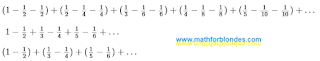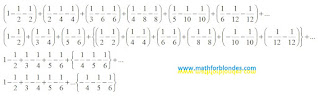## 8/12/2022

### Permutation of terms in infinite sums

From the school bench, everyone knows the rule that appeared in antiquity: the amount does not change from the rearrangement of the terms. By default, it is assumed that the number of terms and their value remain unchanged. If we change the number of terms or change the value of at least one of them, there can no longer be any talk of rearranging the terms. In this case, we have moved from one sum to another and these two sums are not connected in any way.

In the article “What is wrong with the rearrangement of terms?” (in Russian) an example of an infinite sum is given, allegedly proving that the final sum depends on the order of addition.Infinite sum

After opening the brackets and reducing the same terms, the result of the addition is different. That's what mathematicians say. Let's consider this example with more careful observance of the rules for writing mathematical expressions.Permutation of terms in infinite sums

The first line contains the original infinite sum, consisting of six brackets - three terms in each bracket. The total number of terms is 18, the total sum of the expression is zero. The entire mathematical expression can be divided into two groups: the visible part of the expression, represented by 18 terms, and the invisible part of the expression, consisting of an infinite number of brackets, three terms in each bracket. These two parts are separated by the three-dots of infinity. Both parts are zero.

The second line shows the same 18 terms after the permutation. The first three pairs of terms will be further presented in the visible part of the expression. Curly brackets highlight the compensating group of terms.

In the third line, the six terms of the visible part remain unchanged. The compensating group, after simplifying the expression, is represented by three terms. The final result after rearranging the terms has not changed and is still equal to zero.

The fourth line has nothing to do with mathematics. This is the usual trick of an illusionist who hid the compensating group of terms in the arm of infinity (the invisible part of the expression). The purpose of this trick is to convince gullible viewers of the “truthfulness” of the false statement about the change in the total amount after rearranging the terms. Yes, this is what card cheaters do - they hide the card in their sleeve or take it out of there. In the circus, such a trick is called an illusionist's trick. In legal terms, this is called fraud.

We can also give a cruder example of a permutation of the terms in this expression. If terms with only one sign are shown in the visible part of the expression, the audience will inevitably have a question where the terms with opposite signs have gone, and this will clearly make it difficult to demonstrate the trick.

The permutation of terms in infinite sums clearly demonstrates the principle of communicating vessels. The first vessel is the visible part of the expression. The second vessel is the invisible part of the expression, which includes the compensating group of terms. The three-dots of infinity is the connecting pipe between the vessels. The final sum of the expression is the total volume of liquid in the two vessels. Since in our mathematical example the total sum is equal to zero, then in relation to communicating vessels, we consider the initial total liquid level in the vessels as zero of the relative coordinate system. A change in the number or size of terms in the visible part of the expression will lead to a change in the number or size of terms in the compensating group.

If the last expression is considered without an invisible compensating group of terms, then it will not be the result of a rearrangement of the terms, but a completely different infinite sum, containing only a part of the terms from the original expression and not connected with it in any way. The proof of this fact is the different total sum of the two expressions.